# 978-0123869449 Chapter 5 Part 2

Document Type
Homework Help
Book Title
Authors
Michael F. Modest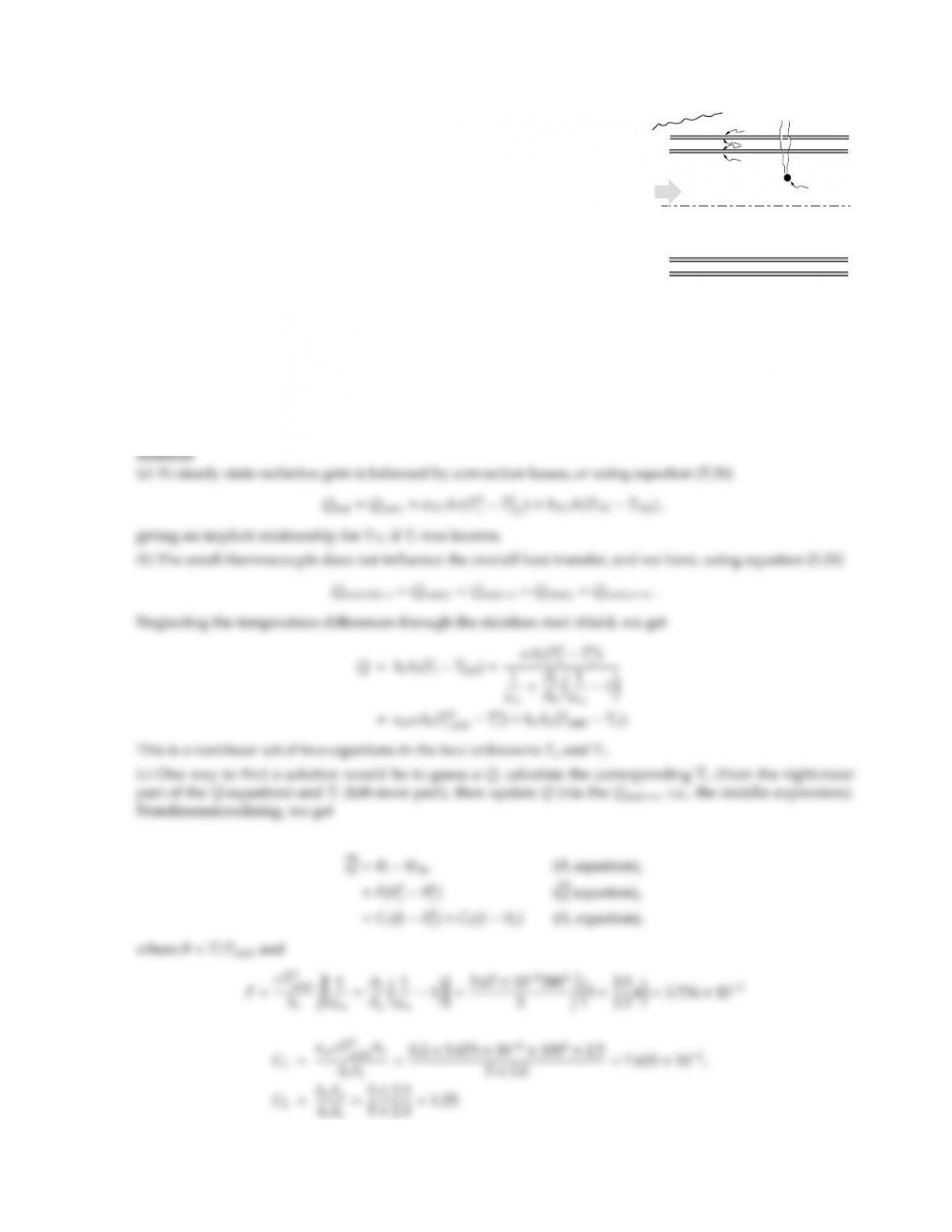5.15
TC
ss
ss, ho
vacuum
helium
ss, hi
TC, hTC
Tamb
A thermocouple used to measure the temperature of cold, low pressure
helium flowing through a long duct shows a temperature reading of
10 K. To minimize heat losses from the duct to the surroundings the
duct is made of two concentric thin layers of stainless steel with an
evacuated space in between (inner diameter di=2 cm, outer diameter
do=2.5 cm; stainless layers very thin and of high conductivity). The
emittance of the thermocouple is ǫTC =0.6, the convection heat transfer
coecient between helium and tube wall is hi=5 W/m2K, thermocouple
and helium is hTC =2 W/m2K, and the emittance of the stainless steel
is ǫss =0.2 (gray and diuse, all four surfaces). The free convection
heat transfer coecient between the outer tube and the surroundings at
Tamb =300 K is ho=5 W/m2K. To determine the actual temperature of the helium,
(a) Prepare an energy balance for the thermocouple.
(b) Prepare an energy balance for the heat loss through the duct wall (the only unknowns here should be
THe and Tw).
(c) Outline how to solve for the temperature of the helium (no need to carry out solution).
(d) Do you expect the thermocouple to be accurate? (hint: check magnitudes of terms in (a)).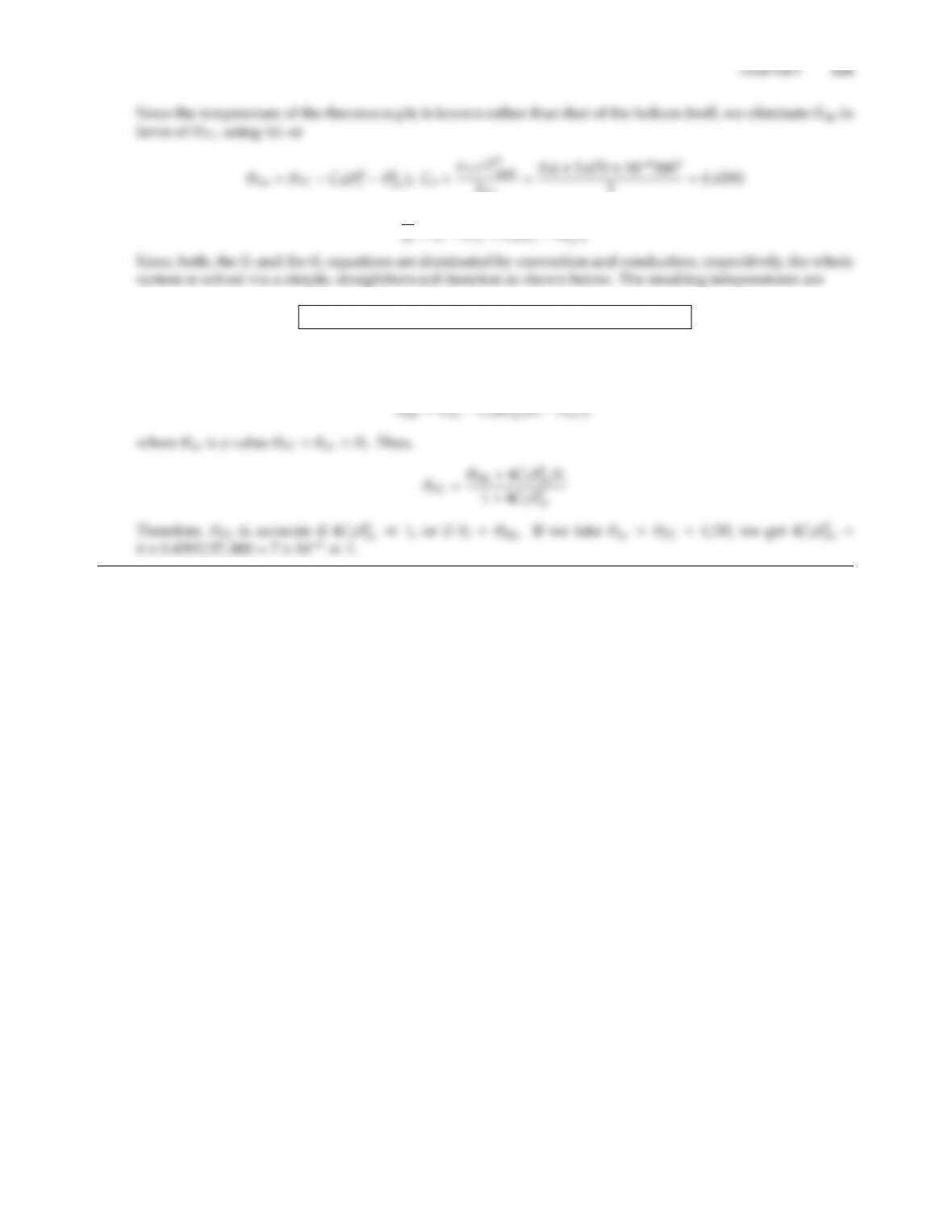hTC
2=0.4593
or
Q=θiθTC +C3(θ4
THe =9.997 K, TTC =10 K, Ti=20.24 K, and To=293.38 K
.
(d) Linearizing the θHe equation, we obtain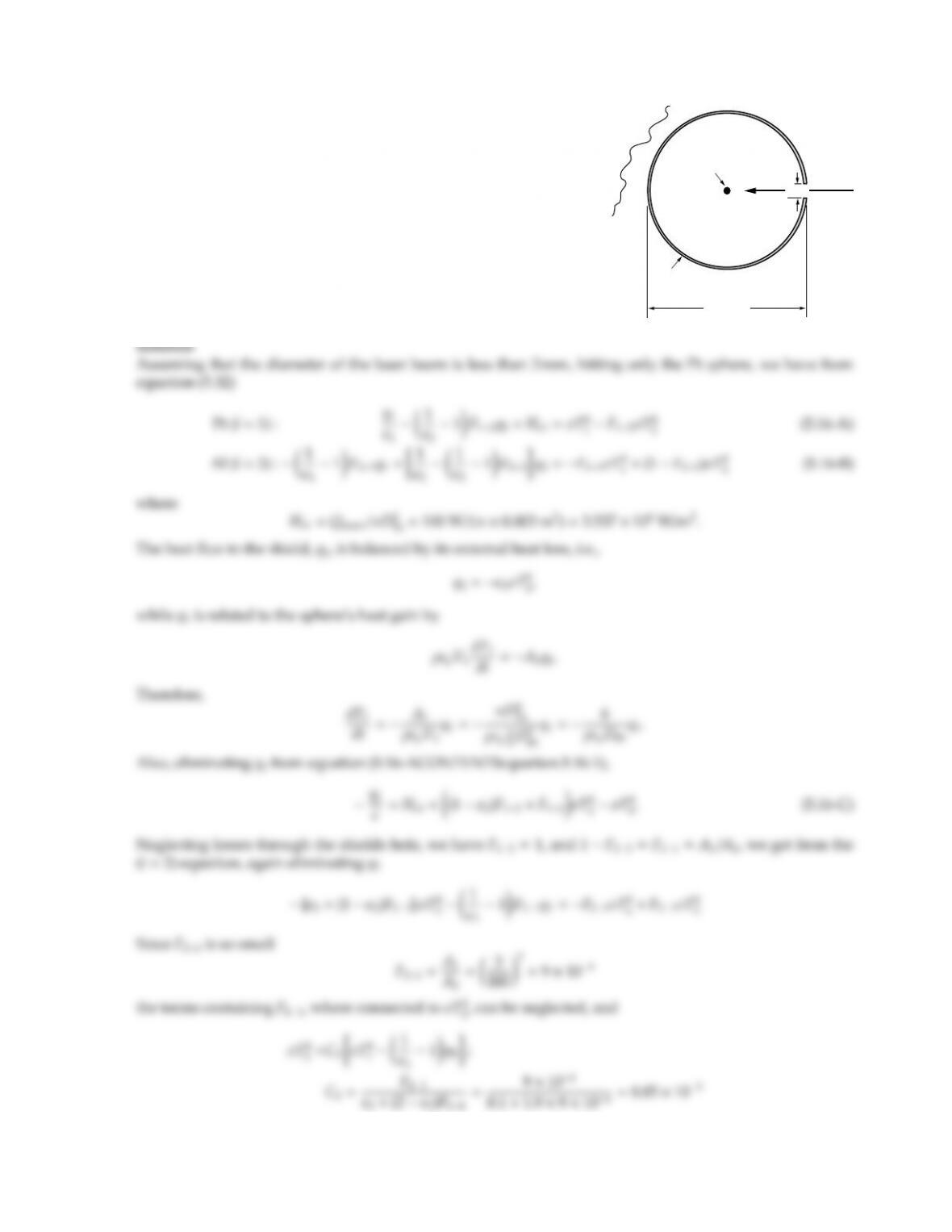5.16
10 cm
0
K
Al shield
Pt sphere
laser
1
cm
During a materials processing experiment on the space shuttle (un-
der microgravity conditions) a platinum sphere of 3 mm diameter
is levitated in a large, cold black vacuum chamber. A spherical
aluminum shield (with a circular cut-out) is placed around the
sphere as shown, to reduce heat loss from the sphere. Initially, the
sphere is at 200 K and is suddenly irradiated with a laser providing
an irradiation of 100 W (normal to beam) to raise its temperature
rapidly to its melting point (2741 K).
Determine the time required to reach the melting point.
You may assume the platinum and aluminum to be gray and dif-
fuse (ǫPt =0.25, ǫAl =0.1), the sphere to be essentially isothermal
at all times, and the shield to have zero heat capacity.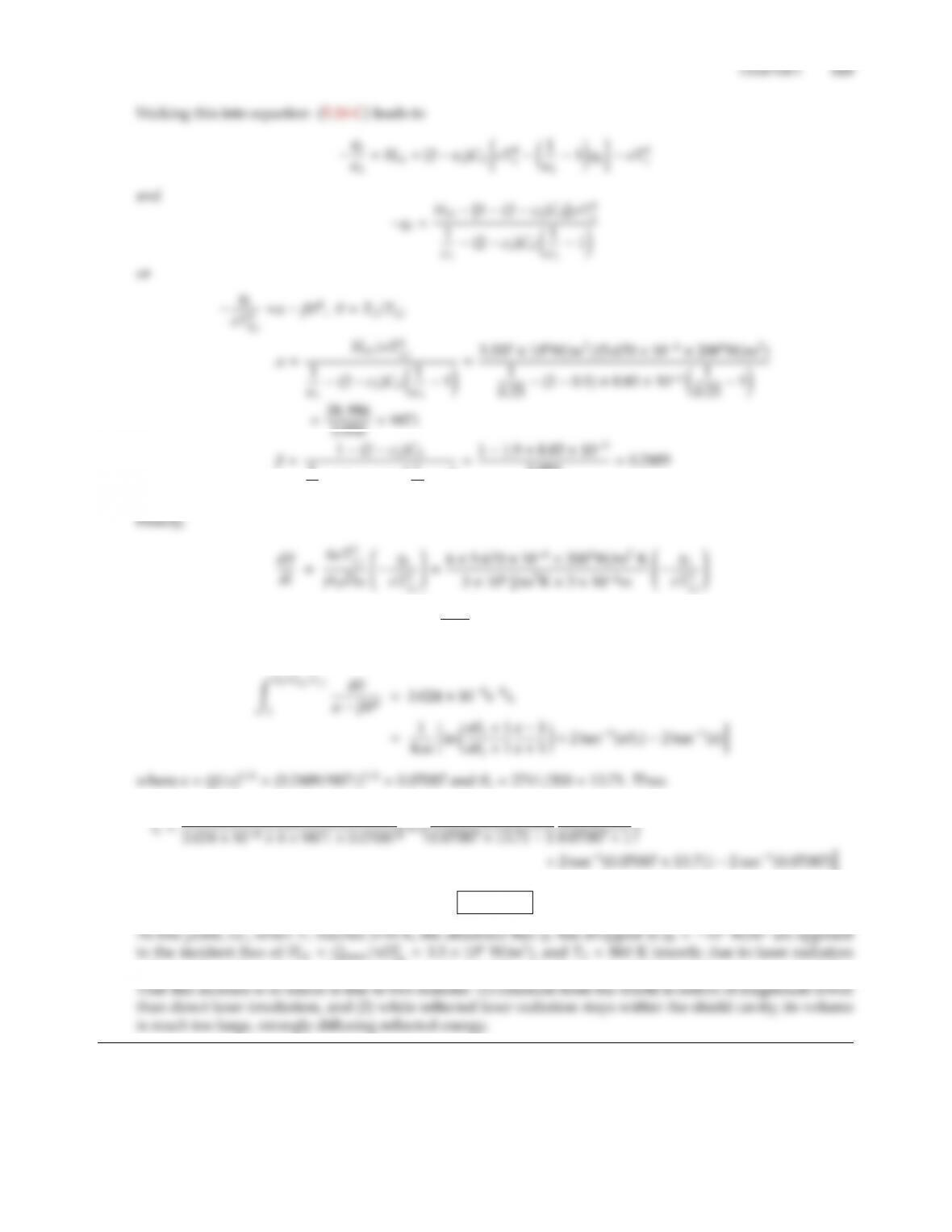1
ǫ1(2 ǫ2)C21
ǫ11=11.9×8.85 ×103
3.950 =0.2489
=3.024 ×104s1q1
σT4
1,i
0.07087 1
te=6.48 s
Pt =3.5×106W/m2), and T2860 K (mostly due to laser radiation
reflected from the sphere). If the shield is neglected (C2=0), the required heating time increases to 6.73 s.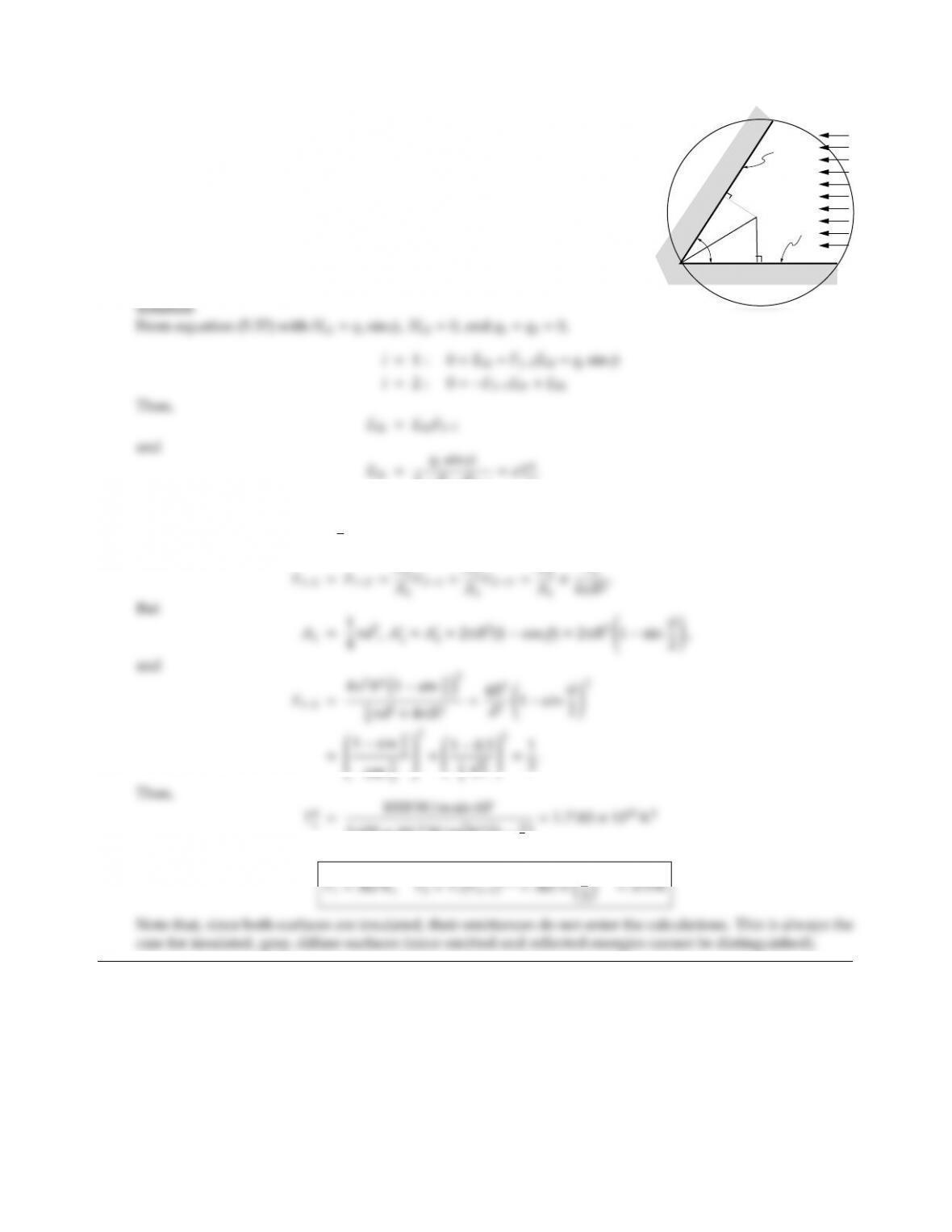5.17
φ
qsun
d
d
A1
A2
R
Two identical circular disks are connected at one point of their periphery
by a hinge. The configuration is then opened by an angle φas shown in
the figure. Assuming the opening angle to be φ=60,d=1 m, calculate
the average equilibrium temperature for each of the two disks, with solar
radiation entering the configuration parallel to Disk 2 with a strength of
qsun =1000 W/m2. Disk 1 is gray and diuse with α=ǫ=0.5, Disk 2 is
black. Both disks are insulated.
1F12F21
1.
The view factor F12=F21is readily determined from the inside sphere method, by placing the disks inside
a sphere of radius R=d/2 cos φ
2(see sketch). Then
cos φ
5.670 ×108W/m2K411
9=1.7183 ×1010 K4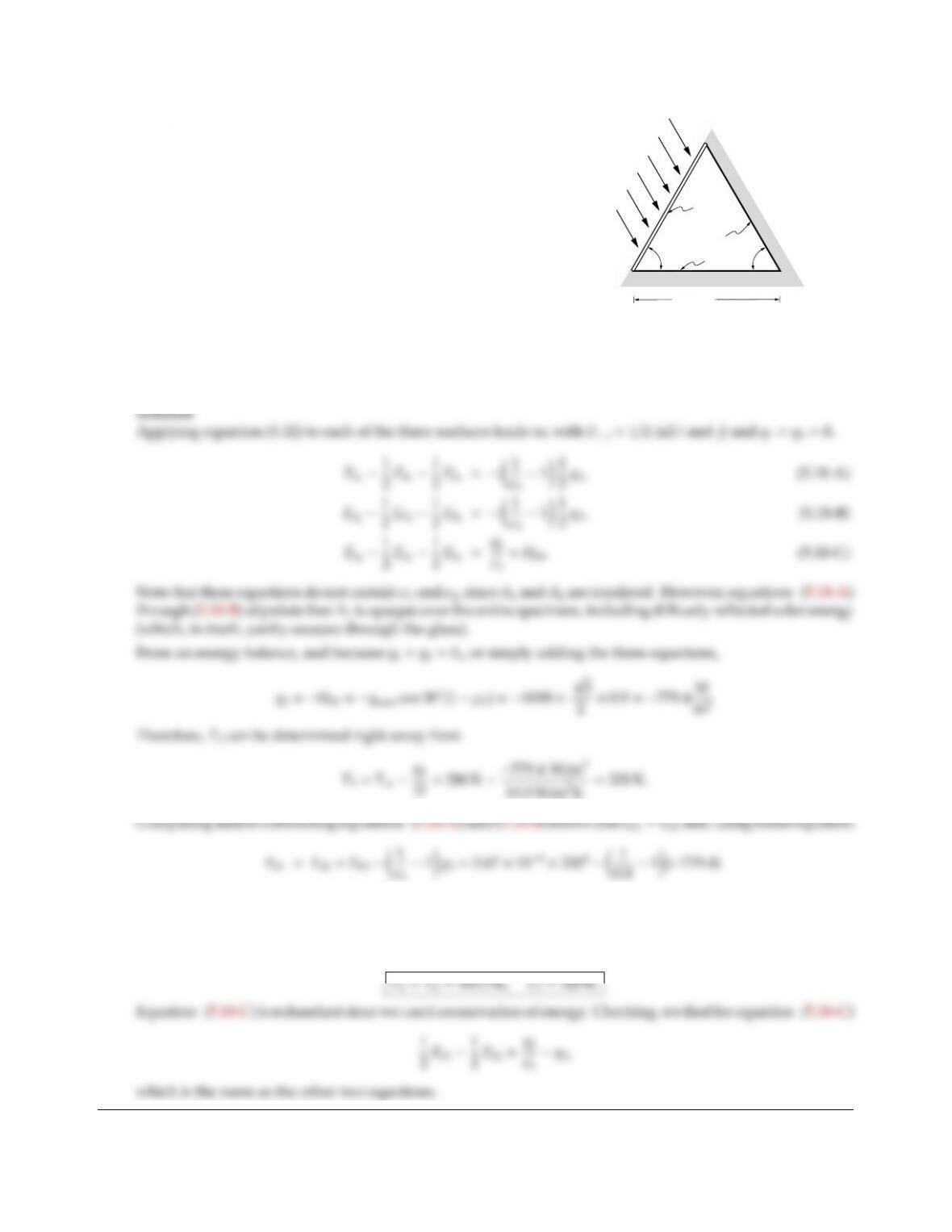CHAPTER 5 125
5.18
60°
A2
A1
A3
60°
L = 1 m
ground
qsun
A long greenhouse has the cross-section of an equilateral triangle
as shown. The side exposed to the sun consists of a thin sheet of
glass (A1) with reflectivity ρ1=0.1. The glass may be assumed
perfectly transparent to solar radiation, and totally opaque to ra-
diation emitted inside the greenhouse. The other side wall (A2) is
opaque with emittance ǫ2=0.2, while the floor (A3) has ǫ3=0.8.
All surfaces reflect diusely. For simplicity, you may assume sur-
faces A1and A2to be perfectly insulated, while the floor loses heat
to the ground according to
q3,conduction =U(T3T)
where T=280 K is the temperature of the ground, and U=
19.5W/m2K is an overall heat transfer coecient.
Determine the temperatures of all three surfaces for the case that the sun shines onto the greenhouse with
strength qsun =1000 W/m2in a direction parallel to surface A2.
=789.4 W/m2
or
T1=T2=789.4/5.67 ×1081/4=343.5 K.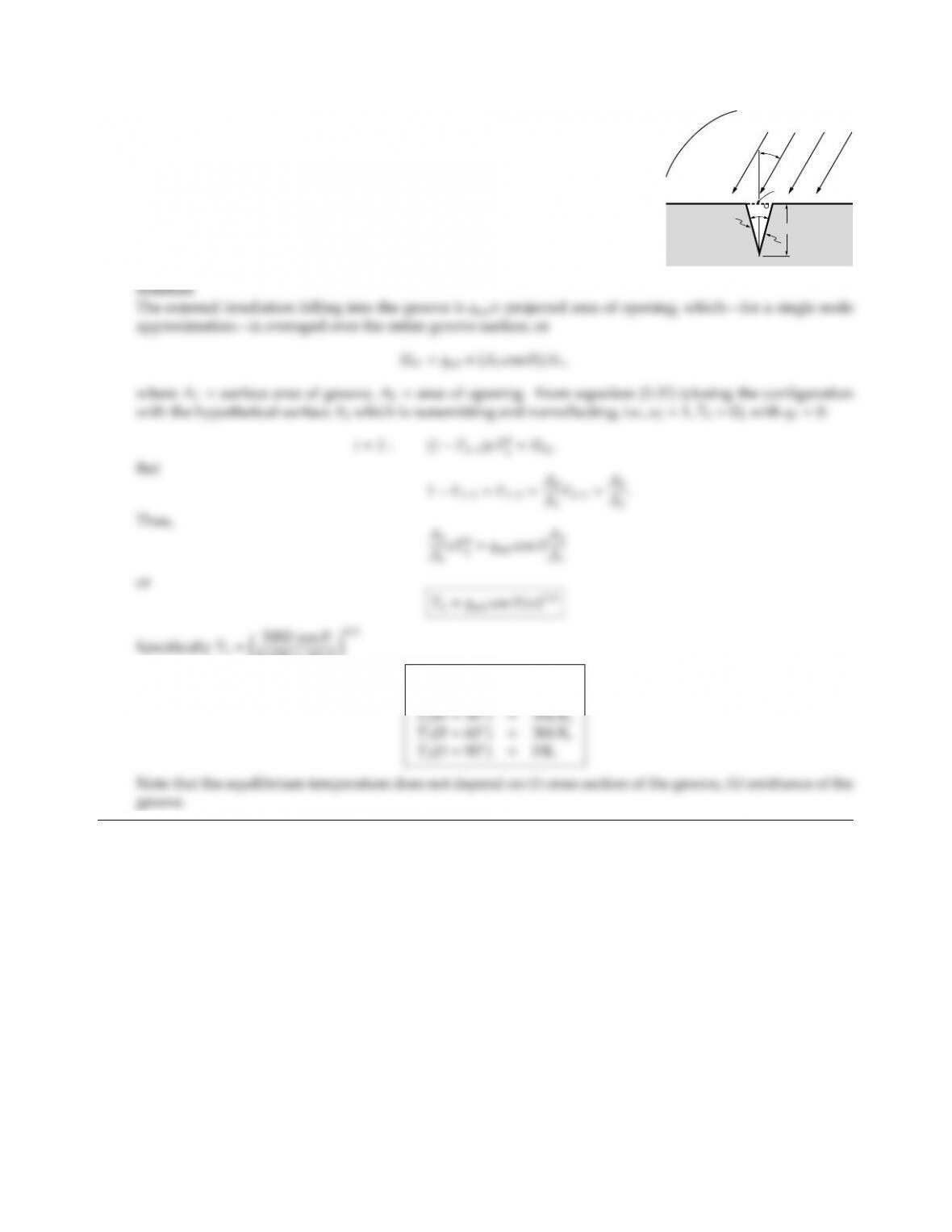5.19
θ
qsol = 1000 W/m2
30
L
0 K
A2
A1
A1
A long, black V-groove is irradiated by the sun as shown. Assuming the
groove to be perfectly insulated, and radiation to be the only mode of
heat transfer, determine the average groove temperature as function of
solar incidence angle θ(give values for θ=0,15,30,60,90). For
simplicity the V-groove wall may be taken as a single zone.
5.670 ×1081/4
T1(θ=0)=364 K,
T1(θ=15)=361 K,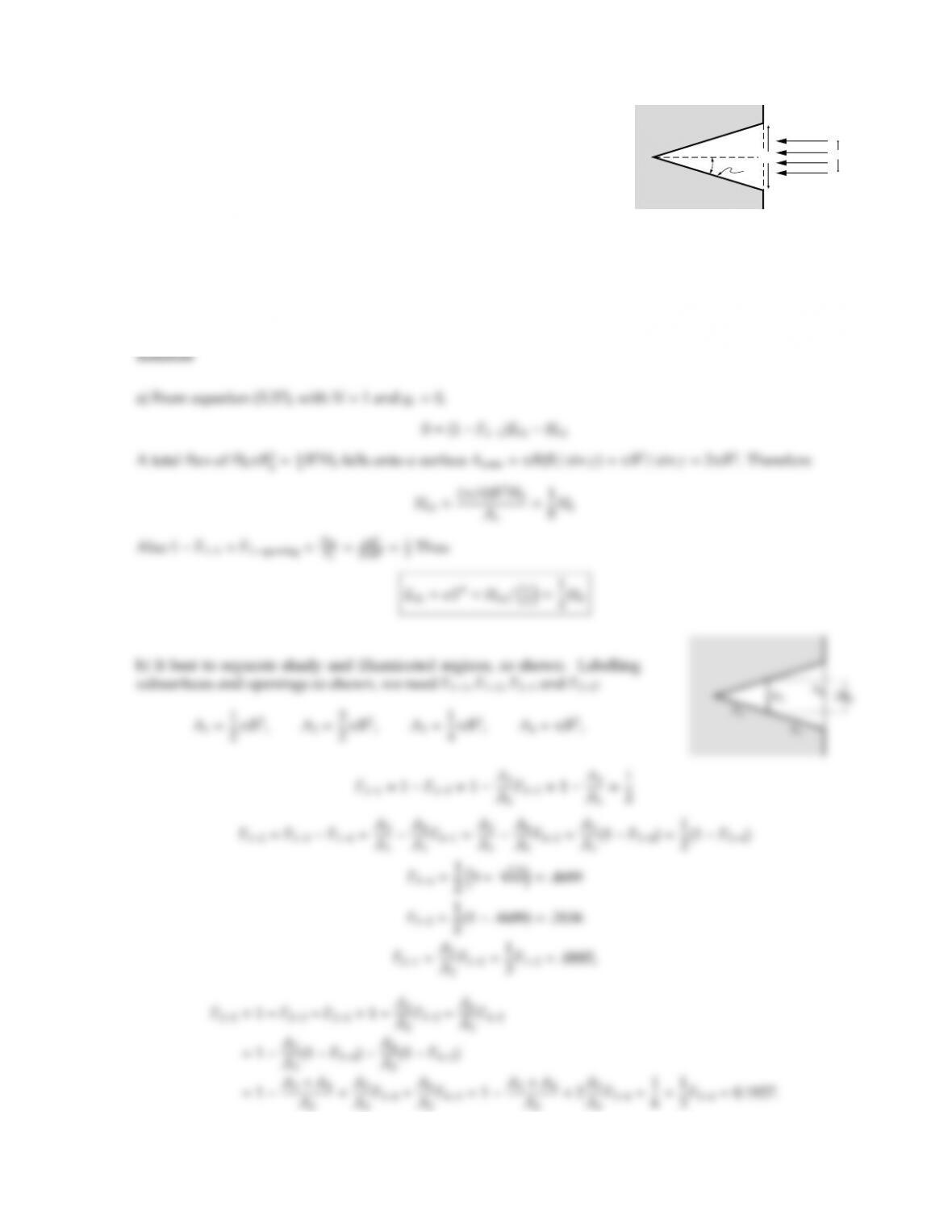CHAPTER 5 127
5.20
Ho
T,
γ
2Rb
2R
Consider the conical cavity shown (radius of opening R, opening angle
γ=30), which has a gray, diusely reflective coating (ǫ=0.6) and is
perfectly insulated. The cavity is irradiated by a collimated beam of
(a) Using a single node analysis, develop an expression relating H0
to the average cavity temperature T.
(b) For a more accurate analysis a two-node analysis is to be performed. What nodes would you choose?
Develop expressions for the necessary view factors in terms of known ones (including those given in
Appendix D) and surface areas, then relate the two temperatures to H0.
(c) Qualitatively, what happens to the cavity’s overall average temperature, if the beam is turned away by
an angle α?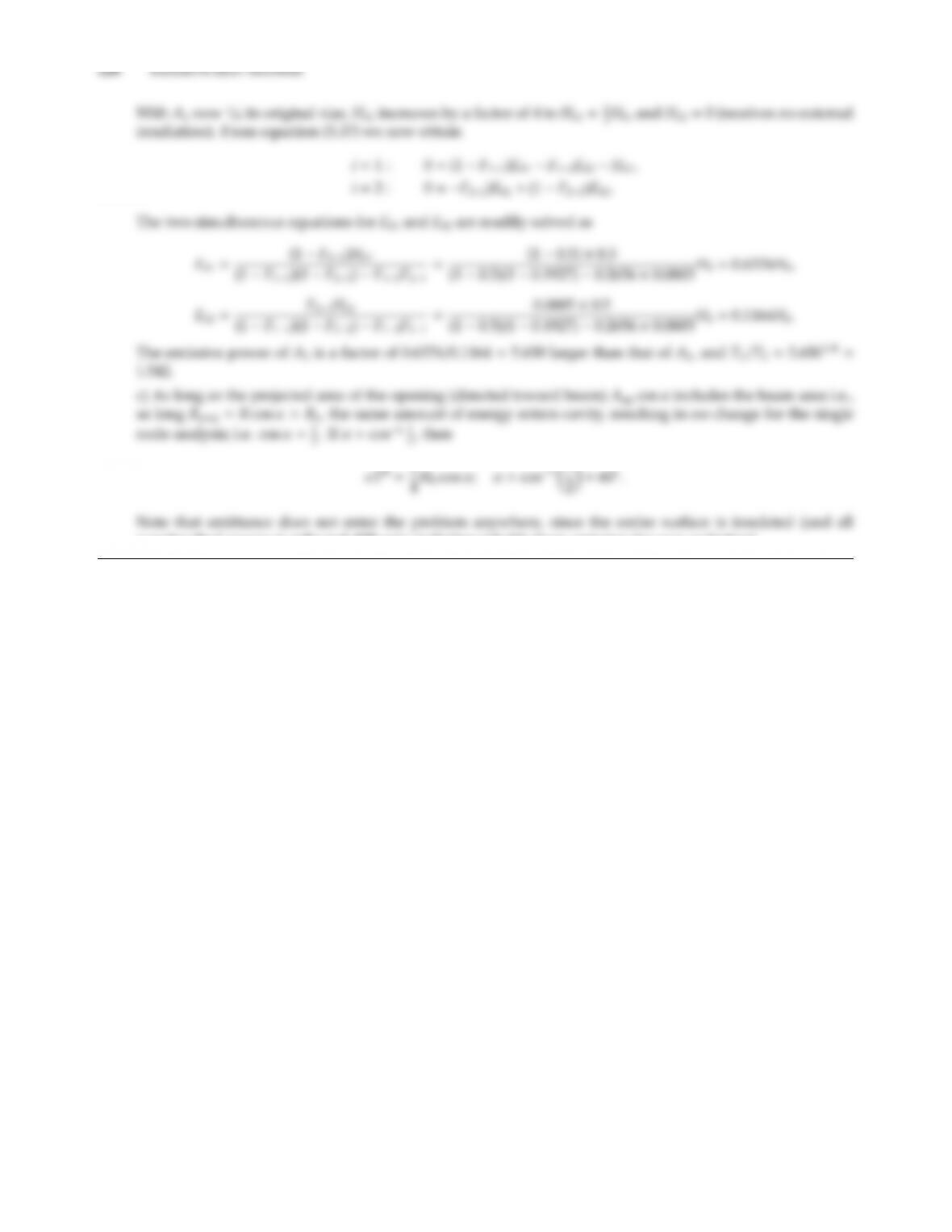nonabsorbed energy is reflected diusely, indistinguishable from emission for gray radiation).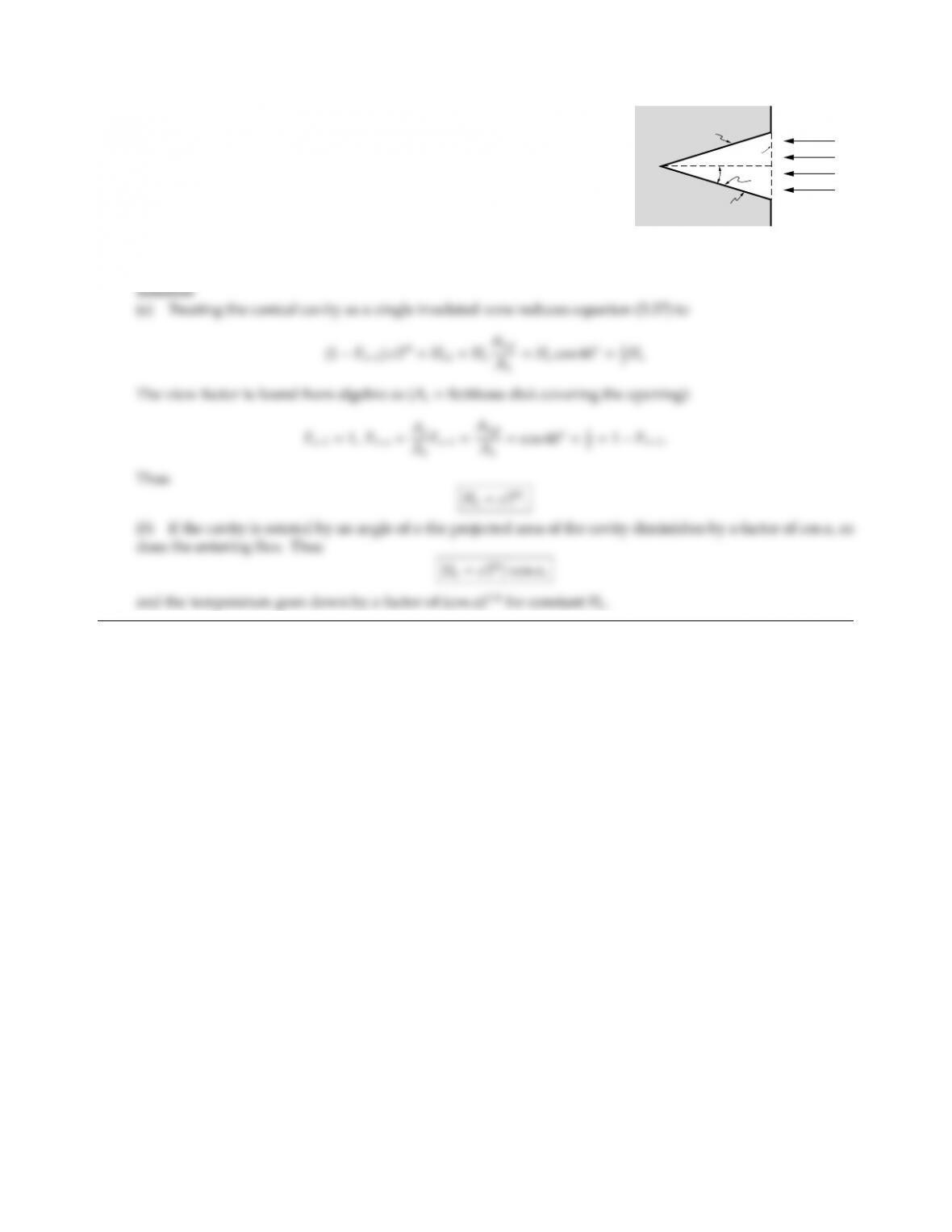CHAPTER 5 129
5.21
Ho
T,
γ
A1
A1
Ae
A (simplified) radiation heat flux meter consists of a conical cavity
coated with a gray, diuse material, as shown in the figure. To measure
the radiative heat flux, the cavity is perfectly insulated.
(a) Develop an expression that relates the flux, Ho, to the cavity
temperature, T.
(b) If the cavity is turned away from the incoming flux by an angle
α, what happens to the cavity temperature?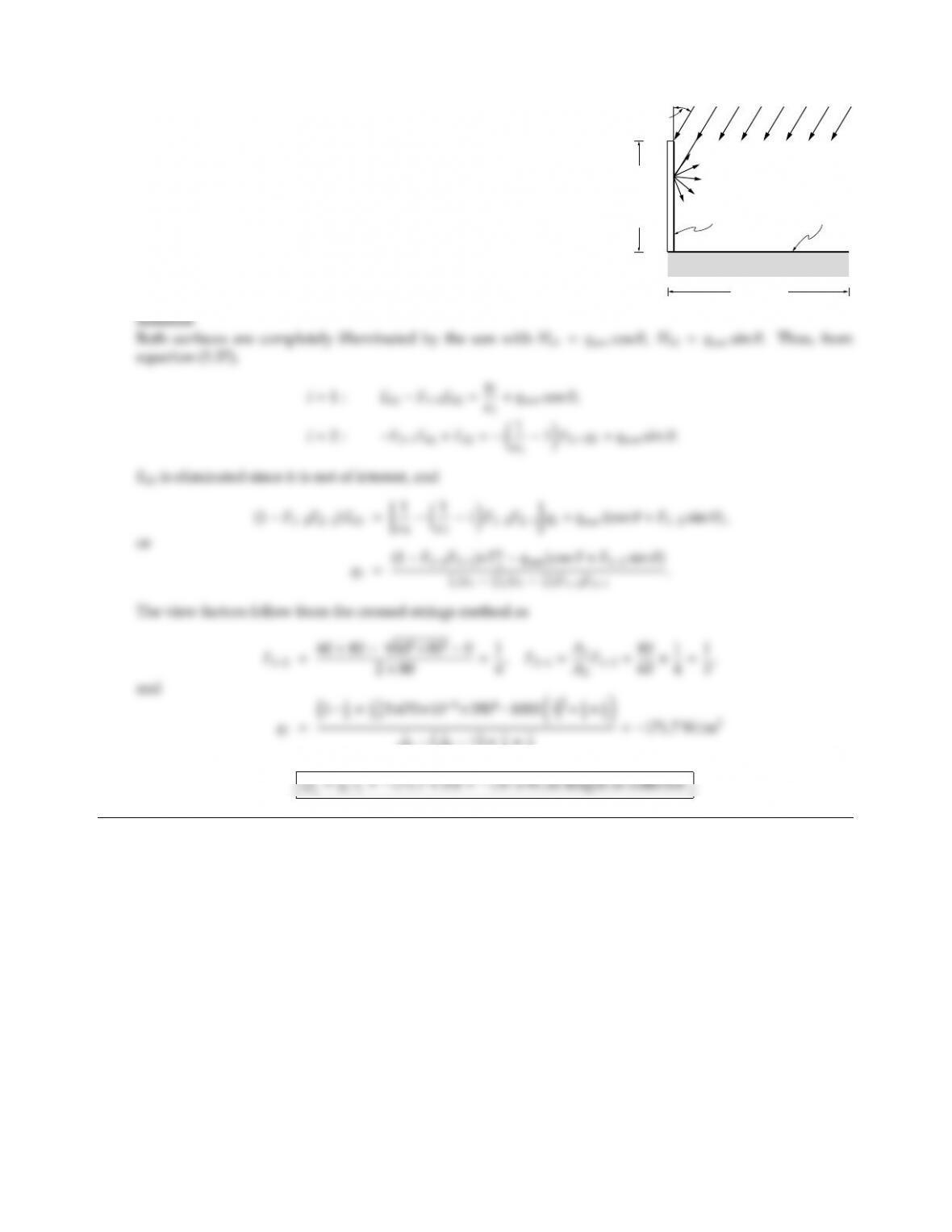5.22
l2 = 60 cm
Reflector
Collector plate
l1 = 80cm
T1, 1
q2 = 0, 2
qsun = 1000 W/m2
30°
A very long solar collector plate is to collect energy at a temperature
of T1=350 K. To improve its performance for o-normal solar in-
cidence, a highly reflective surface is placed next to the collector as
shown in the adjacent figure. How much energy (per unit length)
does the collector plate collect for a solar incidence angle of 30? For
simplicity you may make the following assumptions: the collector
is isothermal and gray-diuse with emittance ǫ1=0.8; the reflec-
tor is gray-diuse with ǫ2=0.1, and heat losses from the reflector
by convection as well as all losses from the collector ends may be
neglected.
0.81
0.81×1
4×1
3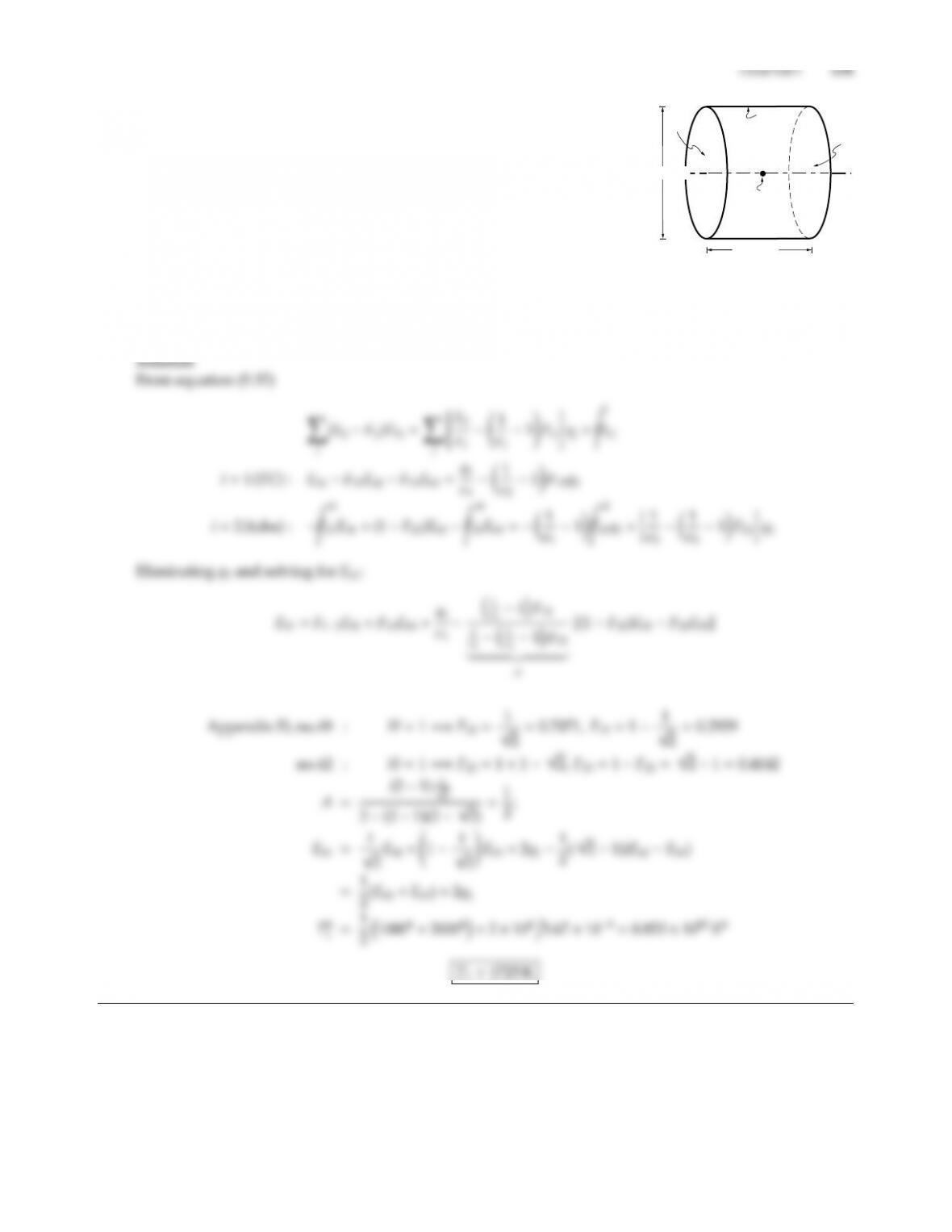5.23
A2
A3
A3
TC(A1)
L=10 cm
D=10 cm
A thermocouple (approximated by a 1 mm diameter sphere with gray-
diuse emittance ǫ1=0.5) is suspended inside a tube through which
a hot, nonparticipating gas at T1=2000 K is flowing. In the vicinity
of the thermocouple the tube temperature is known to be T2=1000 K
(wall emittance ǫ2=0.5). For the purpose of this problem you may
assume both ends of the tube to be closed with a black surface at the
temperature of the gas, T3=2000 K. Again, for the purpose of this
problem, you may assume that the thermocouple gains a heat flux of
104W/m2of thermocouple surface area, which it must reject again in
the form of radiation. Estimate the temperature of the thermocouple.
Hints:
(a) treat the tube ends together as a single surface A3;
(b) note that the thermocouple is small, i.e., Fx11.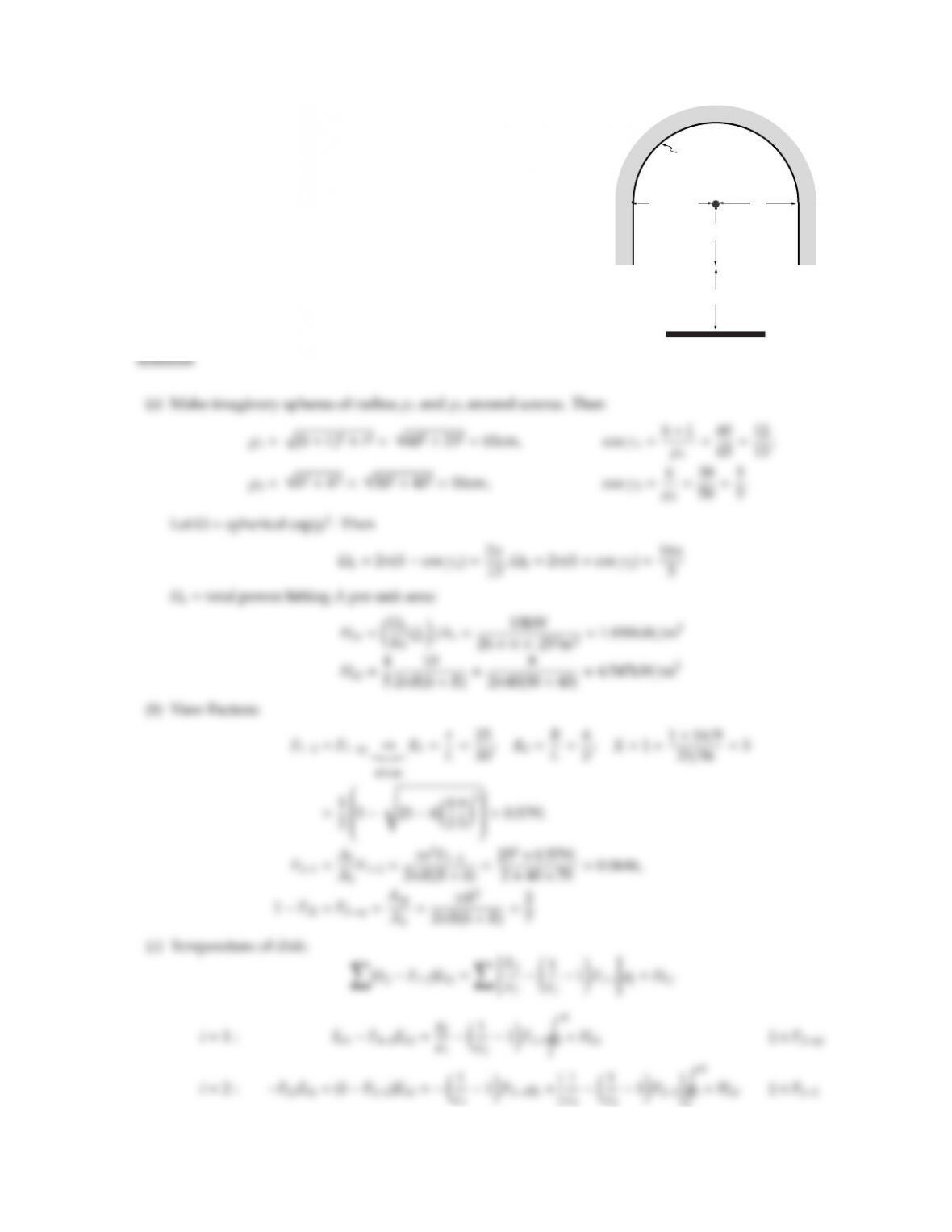5.24
R = 40 cm R
A2: 2 = 0.1, q2 = 0
r=25 cm
h=30 cm
L=30 cm
A1: 1 = 0.8, Q1 = -0.4 kW
Q
s
=10 kW
A small spherical heat source outputting Qs=10 kW power,
spreading equally into all directions, is encased in a reflector as
shown, consisting of a hemisphere of radius R=40 cm, plus a ring
of radius Rand height h=30 cm. The arrangement is used to heat
a disk of radius =25 cm a distance of L=30 cm below the reflector.
All surfaces are gray and diuse, with emittances of ǫ1=0.8 and
ǫ2=0.1. Reflector A2is insulated.
(a) Determine (per unit area of receiving surface) the irradiation
from heat source to reflector and to disk;
(b) all relevant view factors;
(c) the temperature of the disk, if 0.4 kW of power is extracted
from the disk.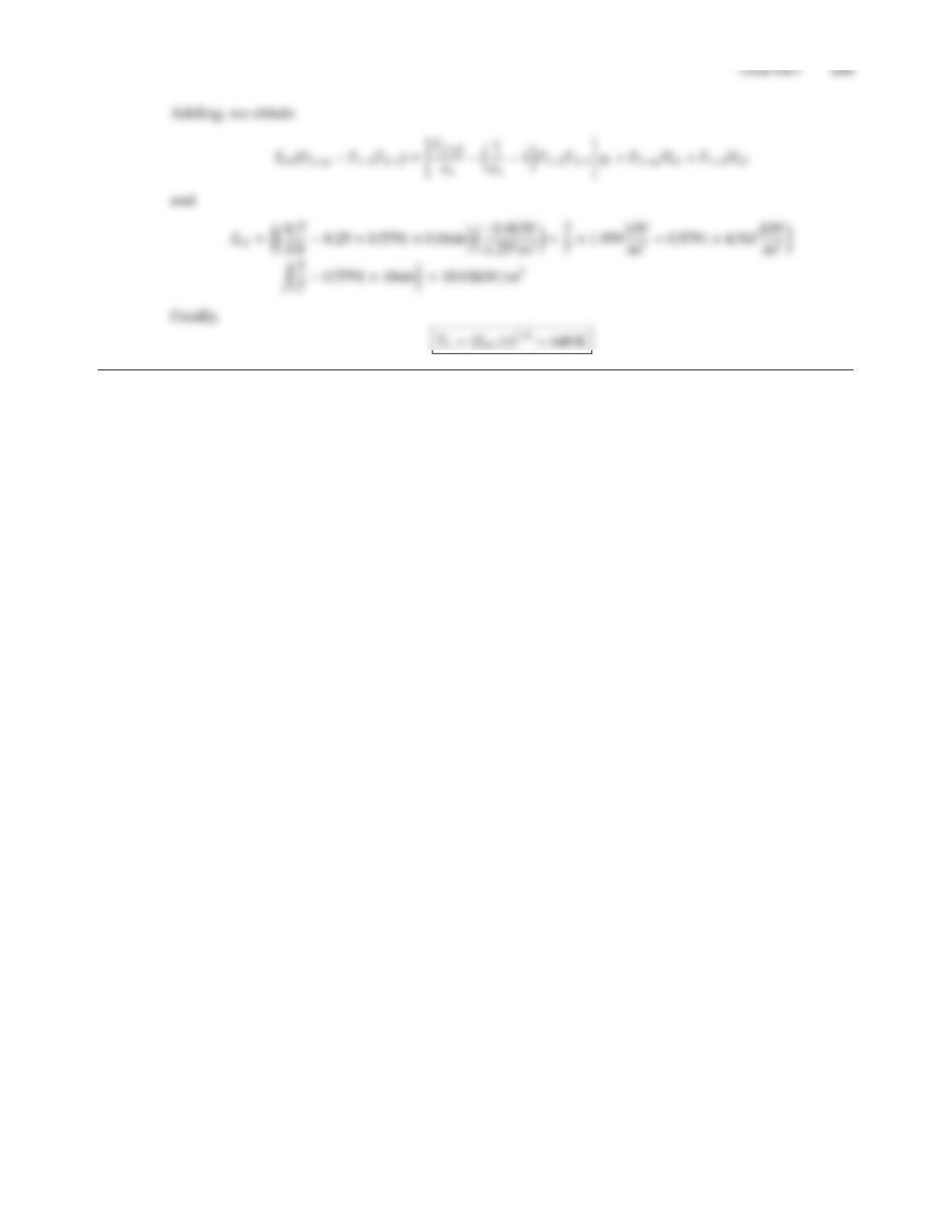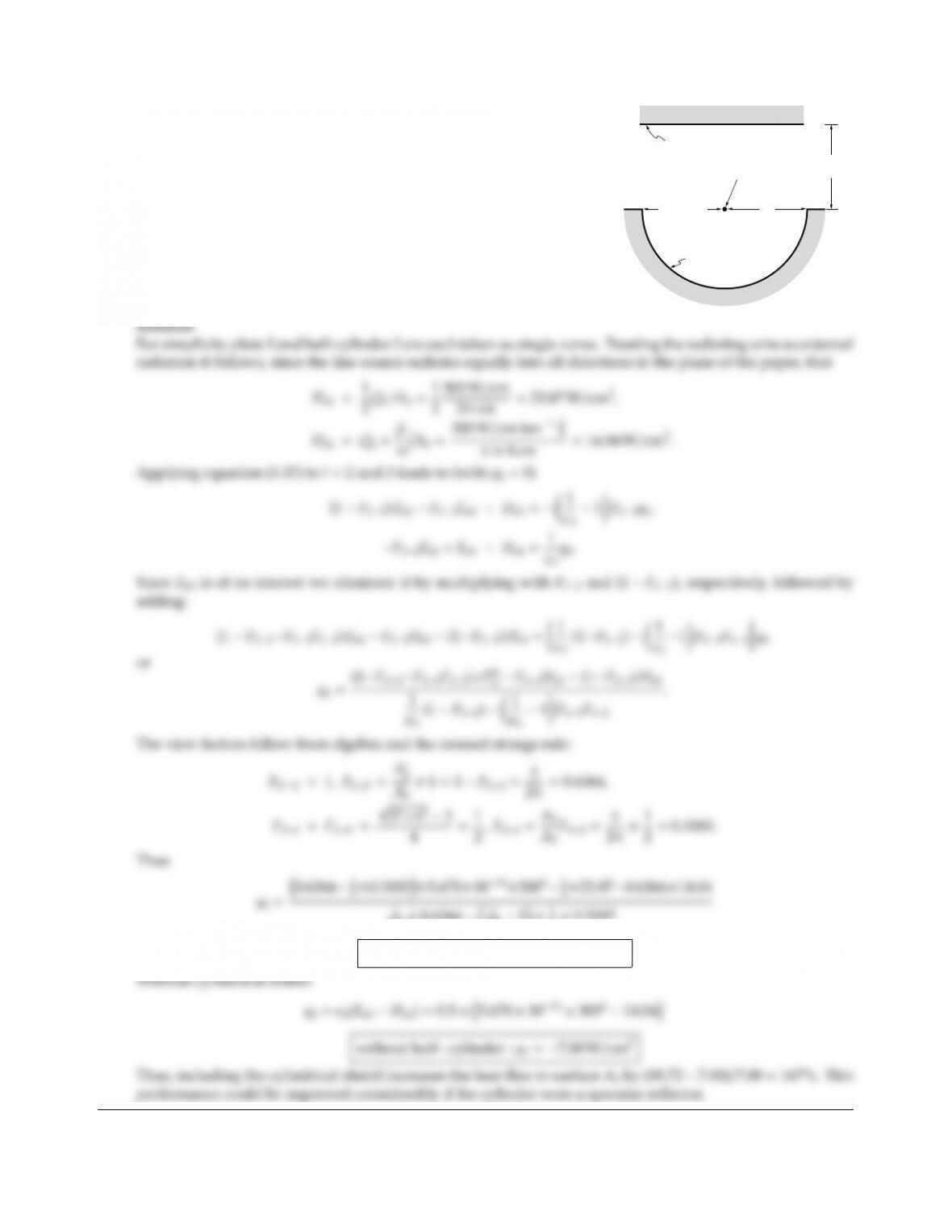5.25
A1: wire
A3: 3 = 0.5, T3 = 300K
R = 2 cm R
h = 3 cm
A2: 2 = 0.2, q2 = 0
A long thin black heating wire radiates 300 W per cm length of
wire and is used to heat a flat surface by thermal radiation. To
increase its eciency the wire is surrounded by an insulated half-
cylinder as shown in the figure. Both surfaces are gray and diuse
with emittances ǫ2and ǫ3, respectively. What is the net heat flux at
Surface 3? How does this compare with the case without cylinder?
Hint: You may either treat the heating wire as a thin cylinder whose
wire as external radiation (the second approach being somewhat
simpler).
0.5×0.6366 1
0.51×1
2×0.3183
with halfcylinder : q3=18.72 W/cm2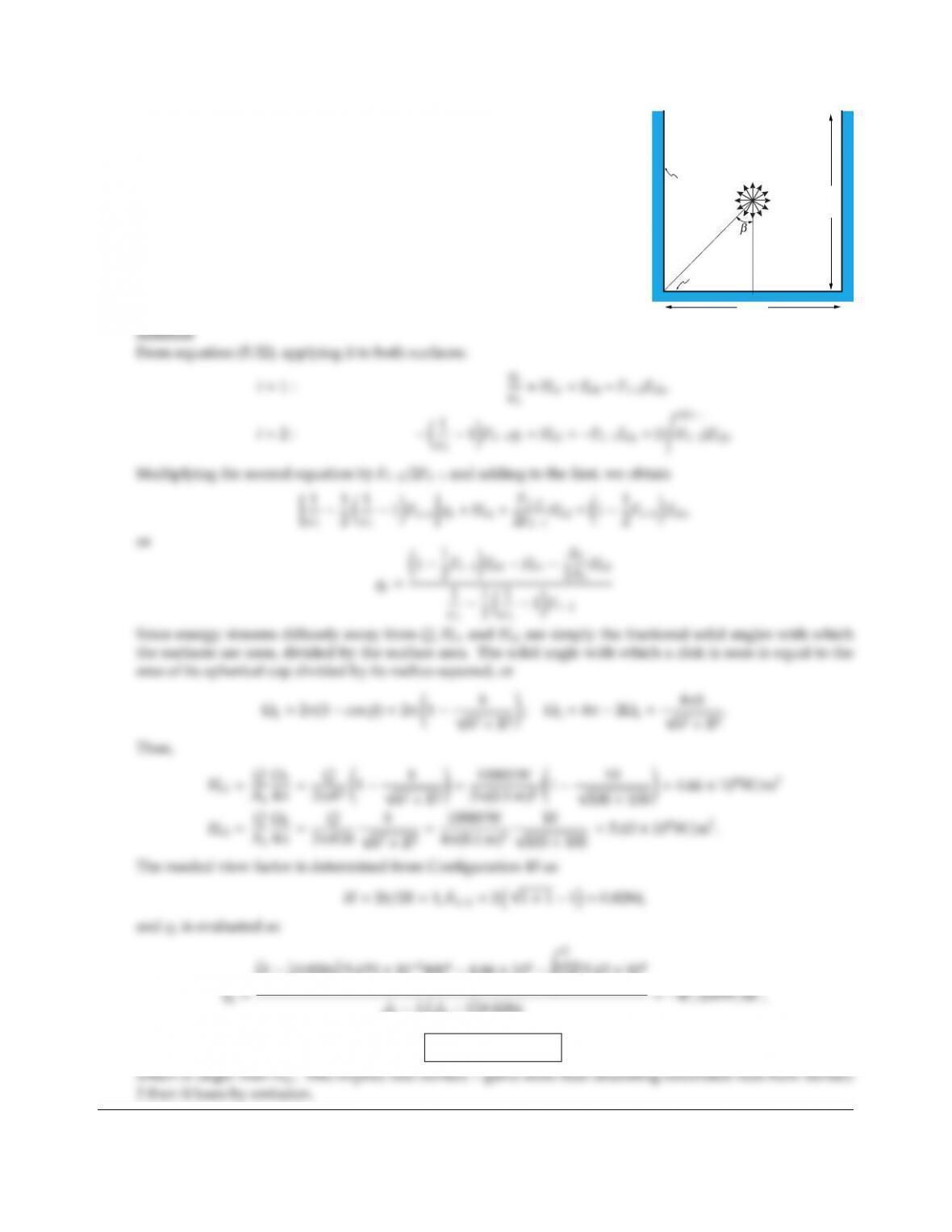CHAPTER 5 135
5.26
h
Q2h
A2
A1
2R
Consider the configuration shown, consisting of a cylindrical cavity A2,
a circular disk A1at the bottom, and a small spherical radiation source
(blackbody at 4000K) of strength Q=10,000 W as shown (R=10 cm,
h=10 cm). The cylinder wall A2is covered with a gray, diuse material
with ǫ2=0.1, and is perfectly insulated. Surface A1is kept at a constant
temperature of 400 K. No other external surfaces or sources aect the
heat transfer. Assuming surface A1to be gray and diuse with ǫ1=0.3
determine the amount of heat that needs to be removed from A1(Q1).
20.82845.670 ×10840044.66 ×104,=2
2πR25.63 ×104
0.31
21
0.310.8284
q1=47,200W/m2,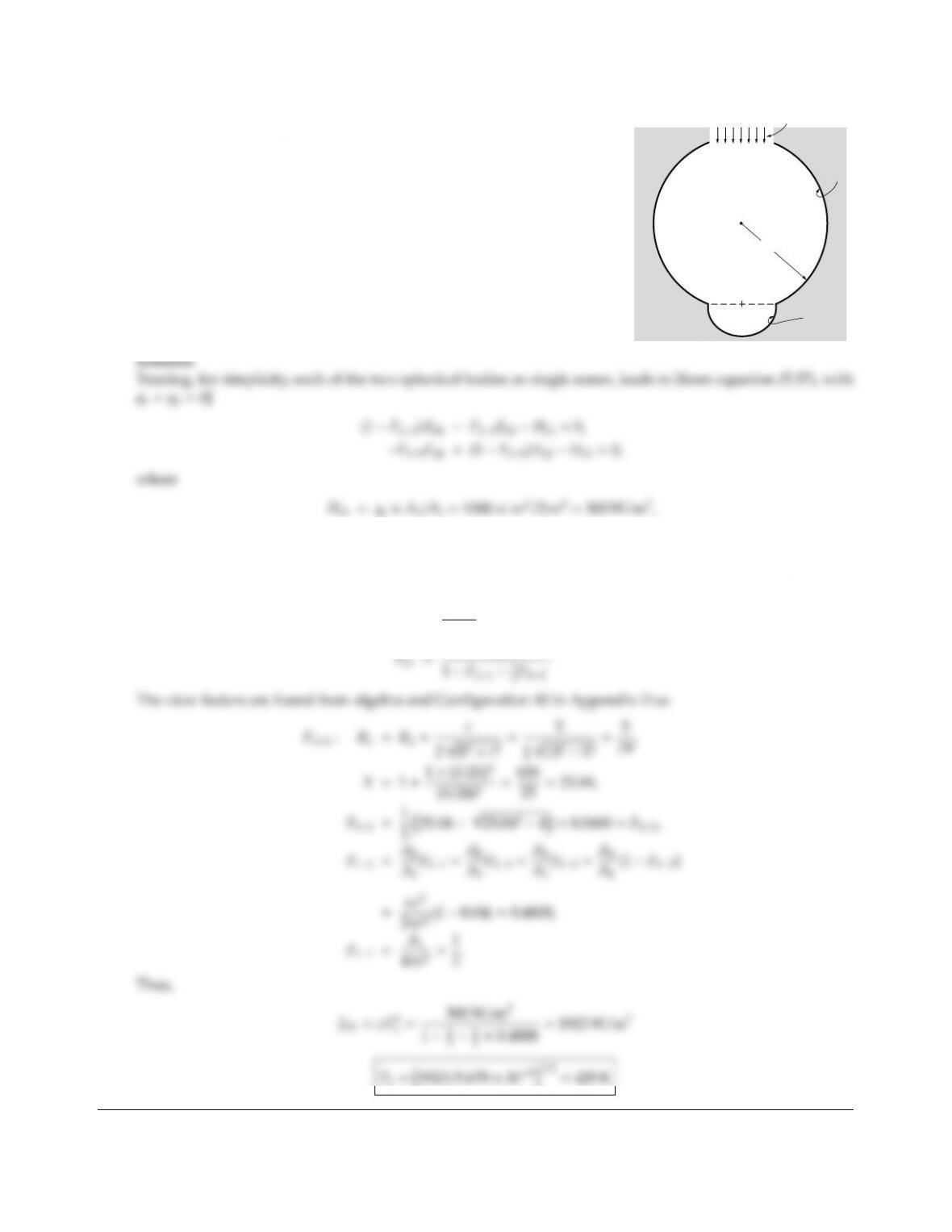5.27
rr
R
qs = 1000W/m2
A2
A1
Determine F12for the rotationally symmetric configuration shown in
the figure (i.e., a big sphere, R=13 cm, with a circular hole, r=5 cm,
and a hemispherical cavity, r=5 cm). Assuming Surface 2 to be gray
and diuse (ǫ=0.5) and insulated and Surface 1 to be black and
also insulated, what is the average temperature of the black cavity if
collimated irradiation of 1000 W/m2is penetrating through the hole
as shown?
Ho2=0.
1F22=F23+F24=2F24=2F21,
Eb2=F21
2F21
Eb1,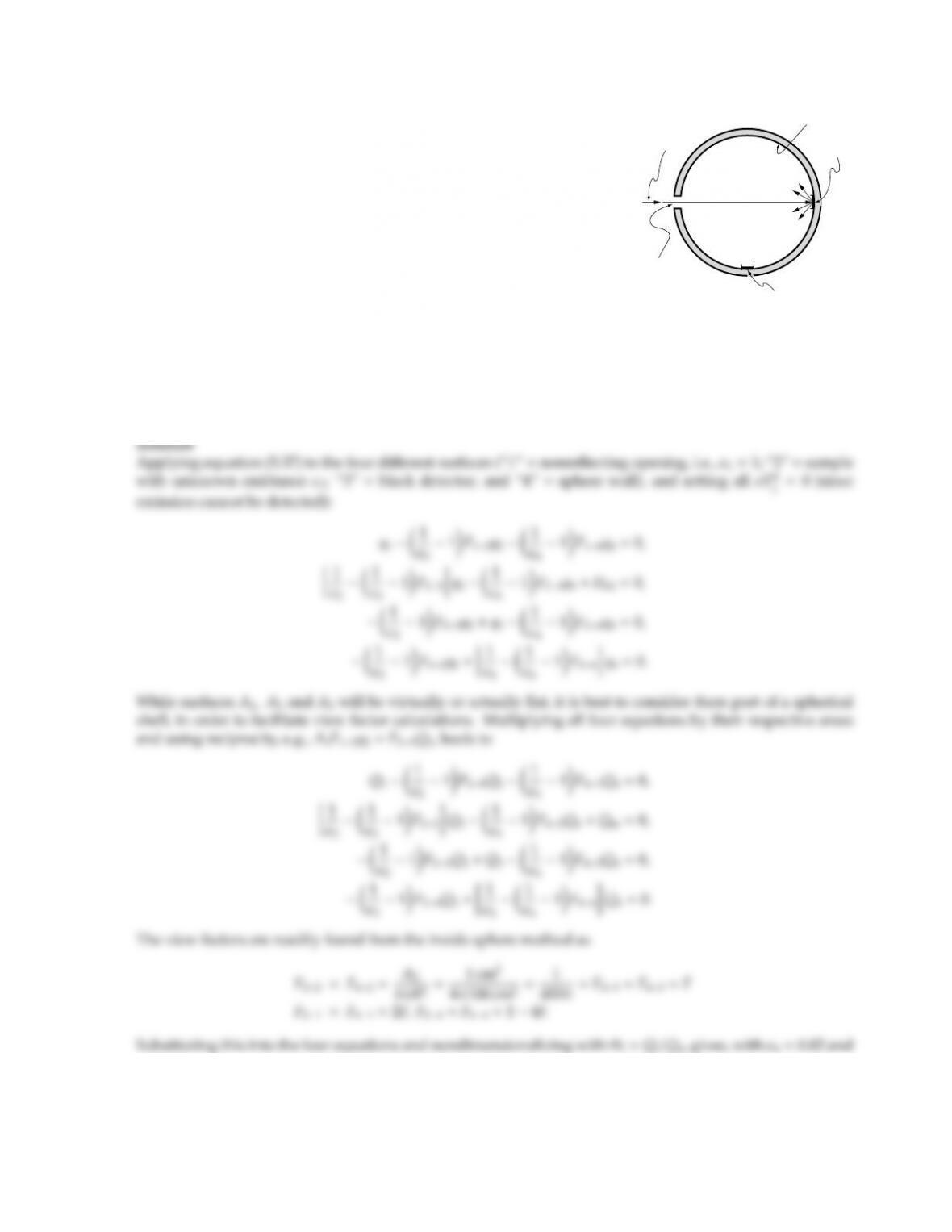CHAPTER 5 137
5.28
Collimated
beam
Entrance
port
Detector
Sample
MgO
An integrating sphere (a device to measure surface properties) is 10 cm
in radius. It contains on its inside wall a 1 cm2black detector, a 1 ×2 cm
entrance port and a 1 ×1 cm sample as shown. The remaining por-
tion of the sphere is smoked with magnesium oxide having a short-
wavelength reflectance of 0.98, which is almost perfectly diuse. A
collimated beam of radiant energy (i.e., all energy is contained within a
very small cone of solid angles) enters the sphere through the entrance
port, falls onto the sample, and then is reflected and interreflected, giv-
from the walls is not detected because the source radiation is chopped,
and the detector-amplifier system responds only to the chopped radi-
ation. Find the fractions of the chopped incoming radiation that are
(a) lost out the entrance port,
(b) absorbed by the MgO-smoked wall, and
(c) absorbed by the detector.
[Item (c) is called the “sphere eciency.”]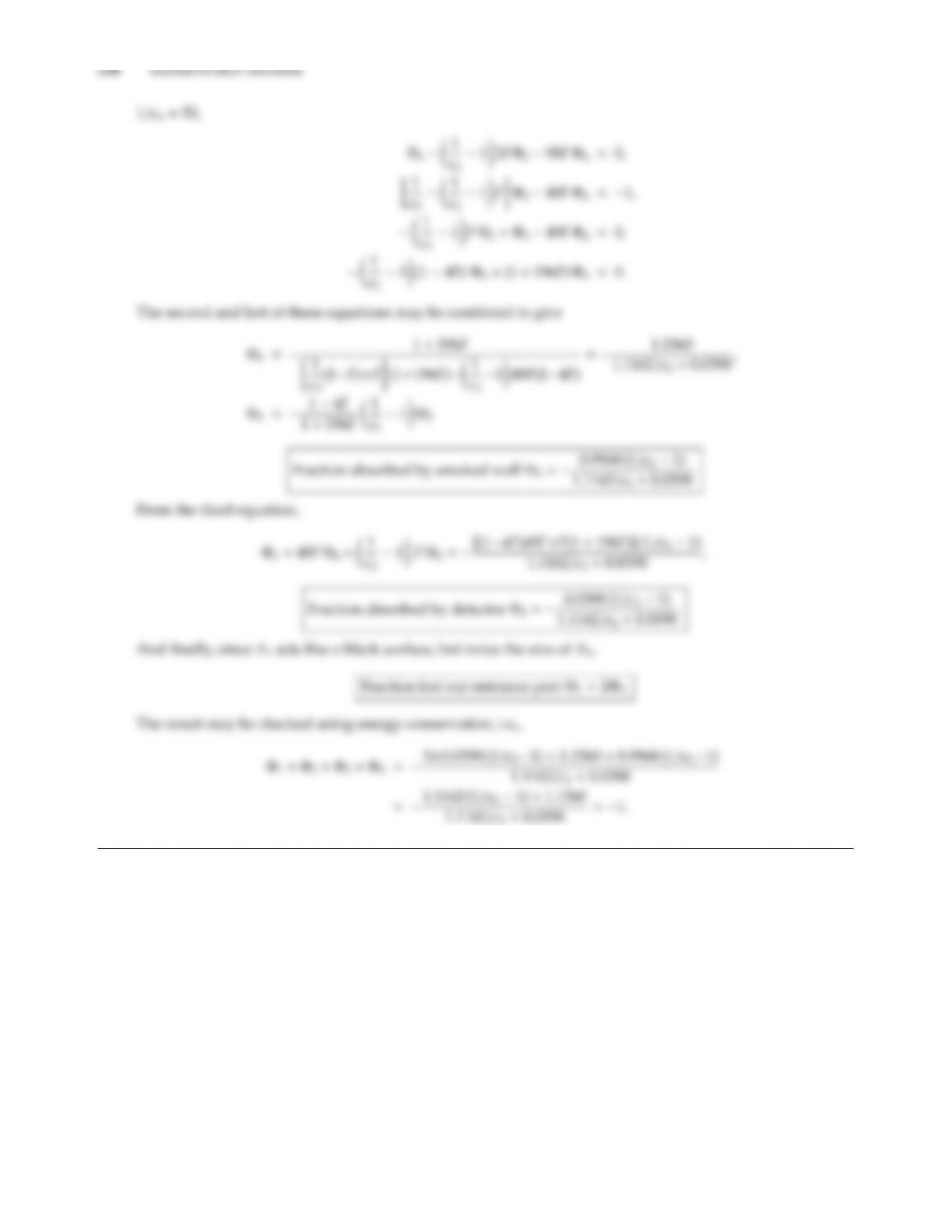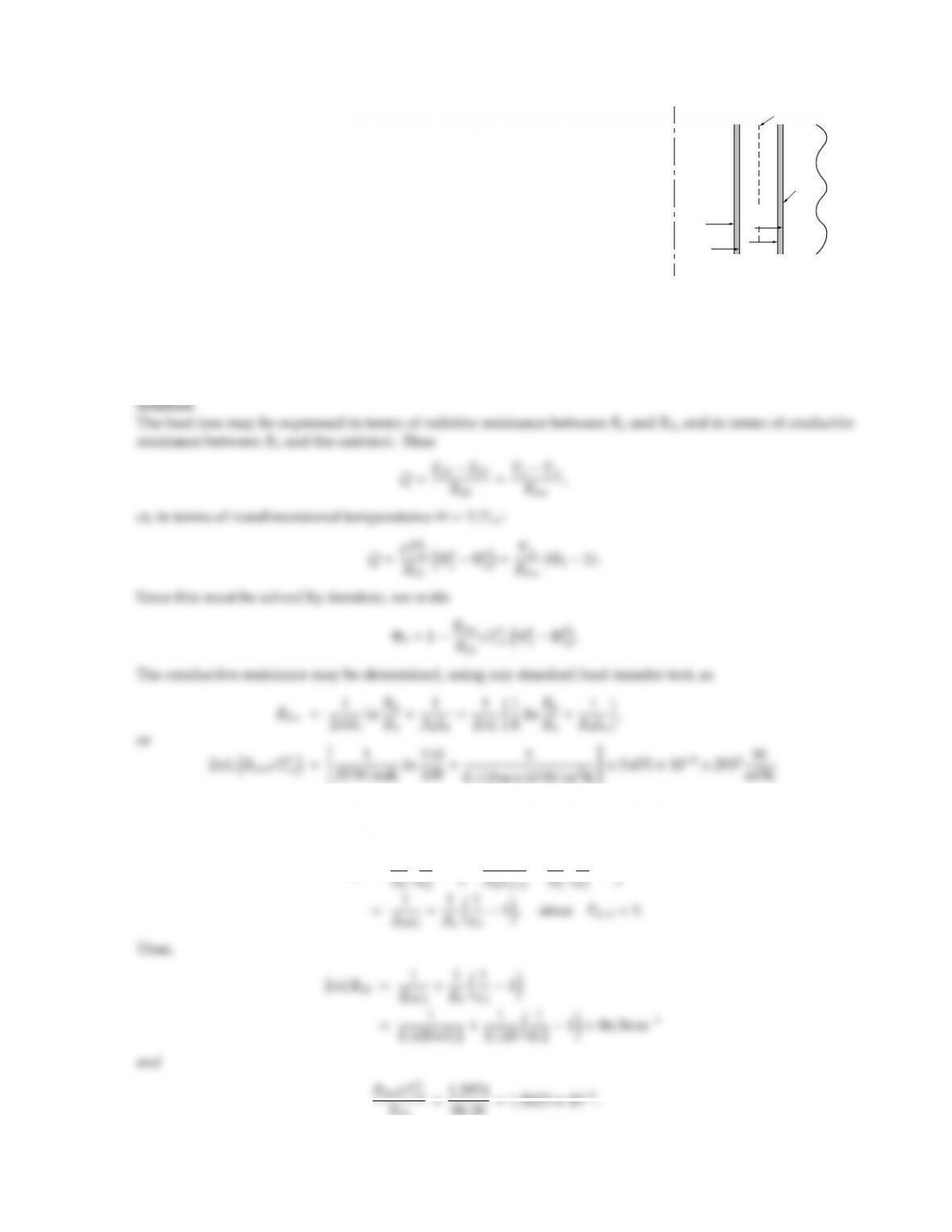CHAPTER 5 139
5.29
R2
Liquid
helium
Foil
ho
R1
R3
Tamb
R4
The side wall of a flask holding liquid helium may be approximated as
a long double-walled cylinder as shown in the adjacent sketch. The con-
tainer walls are made of 1 mm thick stainless steel (k=15 W/m K, ǫ =0.2),
and have outer radii of R2=10 cm and R4=11 cm. The space between
walls is evacuated, and the outside is exposed to free convection with the
ambient at Tamb =20C and a heat transfer coecient of ho=10 W/m2K
(for the combined eects of free convection and radiation). It is reason-
able to assume that the temperature of the inner wall is at liquid helium
temperature, or T(R2)=4 K.
(a) Determine the heat gain by the helium, per unit length of flask.
(b) To reduce the heat gain a thin silver foil (ǫ=0.02) is placed midway between the two walls. How does
this aect the heat flux?
For the sake of the problem, you may assume both steel and silver to be diuse reflectors.
=1.2974 m1.
(a) Without the silver foil the radiative resistance follows from Fig. 5-10cas
R23 =1
+1
R23
86.36 =1.5023 ×102.

## Trusted by Thousands ofStudents

Here are what students say about us.